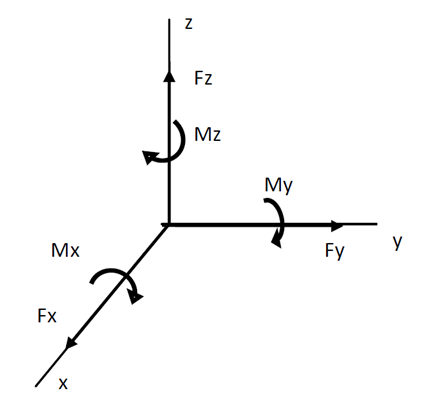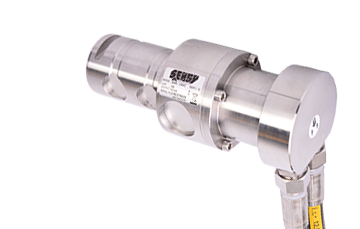# FORCE MEASUREMENT

## Vectorial issues• ### Force definition

In classical mechanics, a force is defined as "an action capable of modifying the quantity of movement of a material point". Therefore, a force has the attributes of a vector and is characterised by an application point, a direction and an intensity. Moreover, the set of forces applied to a point can be reduced to a system composed of three mutually orthogonal forces Fx–Fy–Fz and three moments Mx–My–Mz on these three axes. This group is known as the force torsor applied on this point.

• ### Force torsor diagram

This vectorial issue makes force and torque measurement a complicated matter. In practice, unidirectional and isolated pure forces do not exist. Other forces and moments always overlap and interfere with the force to be measured. That’s why precautions must be taken to eliminate them. To summarise, two characteristics – application point and direction – must be determined in order to measure a force, before applying techniques and methods to measure its intensity.

It is equally useful to specify that a body at rest is subject to a set of forces and torques yielding a zero result.

## Action and reaction

This very same mechanism demonstrates the action and reaction principle, whereby "if a force is exerted on material point P2 by material point P1, then P2 will apply an equal force on P1 in the opposite direction". This is an important principle for the measurement of force. Thus, it is necessary to take this other force into account, as well as the way it acts on the force measurement instrument, since it can have a significant impact on measurement.## Isostatism

Isostatism refers to the contact between two solids with a strictly minimum number of contact points. This simplifies the system of forces and moments involved in the contact and makes it possible to identify them. It therefore allows for better control of the system of forces present, resulting in better measurements.

## Action-at-a distance

The elasticity of materials theory concluded that the further away strain deformations are located from the point where such strain is applied, the more linear the deformation will be. In other words, the greater the accuracy sought, the greater the dimensions of a force measurement instrument fitted with strain gauges have to be.

NOTE : Spanish version /en/tecnologia/sensores-de-fuerza/medicion-de-la-fuerza